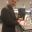# π vs τ: FOTSN/Tau Day special

Since it’s $\tau$ Day, we thought we’d give Festival of the Spoken Nerd constant-fans Matt Parker and Steve Mould a chance to air their respective viewpoints in the $\tau$ vs $\pi$ debate. It’s a maths showdown!Steve Mould

$\pi$ is the circumference divided by the diameter. The diameter! What a strange choice. In maths we prefer to talk about the radius. $\pi$ enthusiasts say that the diameter is the only thing you can directly measure. But how often does our use of $\pi$ begin with the physical measurement of a circle? And how often do we use the diameter in our equations? Engineers might say “often”. For everyone else, setting the circle constant to the circumference divided by the radius is conceptually clearer. So let’s say $\tau=2\pi$ and start using that instead.

There are $2\pi$ radians in $1$ whole turn. With $\tau$ you get $1 \tau$ radians in $1$ whole turn. Much easier.

But what about Euler’s identity? Well that becomes:

$e^{i\tau} = 1$

Beautiful. And look at these:

\begin{align} e^{i \frac{\tau}{4}} &= i \\ e^{i \frac{\tau}{2}} &= -1 \\ e^{i \frac{3 \tau}{4}} &= -i \\e^{i\tau} &= 1 \end{align}

See how the factor of $\frac{1}{4}$ represents $\frac{1}{4}$ of a turn through the complex plane and so on. With $\pi$ that’s hidden.

The area of a circle becomes $\frac{\tau}{2} r^2$. Surely $\pi$ would be better here? Not at all. The half tells us that we’ve performed an integration. With $\tau$, unexpected terms tend to point you in interesting directions. With $\pi$ they’re obscured.

Sticking with $\pi$ is just irrational.Matt Parker

If $\tau$ is so great why did we even have $\pi$ in the first place? Why have we used $\pi$ for millennia instead of switching to $\tau$ if it’s so great? Because from the very first circle-measurements of prehistory to modern precision engineering: the direct measurement you can make of a circle is its diameter, not its radius. Comparing the ratio of diameter to circumference is more fundamental.

$\tau$ evangelists can wheel out as many formulae and equations as they want containing $2\pi$, they are just cherry-picking. There are just as many equations using $\pi$, including the immortal $e^{i\pi} + 1 = 0$. If you convert Euler’s Identity to use $\tau$, you no longer have the irrational exponential constant, the transcendental circle constant, imaginary numbers, unity, negative numbers and zero all linked together in one relationship.

Of course the final word in this argument will come down to radians. $\pi$ radians gives you half a circle, $\tau$ radians gives you a whole circle. $\pi$ is the more nuanced base-unit; it actually divides up what it is measuring. $\tau$ radians will bring you right back to where you started; with $\pi$ radians you actually get somewhere.

Festival of the Spoken Nerd will be appearing at this year’s Edinburgh Festival from 1-7 August, and touring the UK in October and November. For more details, visit FOTSN.com.

•#### Matt Parker

Maths. Stand-up. Whatnot.
•### 2 Responses to “π vs τ: FOTSN/Tau Day special”

1.Colin Beveridge

How about a bar with three legs to represent 2π/3?

2.John Nicholson
With $\tau$ and Euler’s Identity you get to go one step forward (or 1), with $\pi$ you go two steps backward from $\tau$ (or -1).